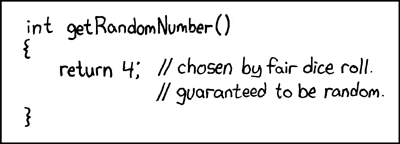The concept of random is pretty interesting when you think about it from a computer's point of view, because without some external input, random is impossible.

Computers (hardware and software) are deterministic. Meaning you give some input and get an output, and if you repeat the input, you'll get the same output. Random numbers are, from a philosophical stand point, indeterministic.

So random functions in software, like `Math.random()` starts with a seed value and roughly speaking, the result of the random function call is used as the next seed, and the next and so on. This means that computers can generate a random sequence of values but to start it needs a seed. A good candidate for a seed is the current time in millisecond for instance.

In "most" cases, these pseudo random values are fine, though if you want to do some in depth reading on random number generation in V8 there's a great article on Medium entitled TIFU by using Math.random() (archived copy).

## Random for games

Lately I've been playing with my own Tetris clone and added an (undocumented) two player option. When the game plays, the tetrominos are selected "at random". However in a two player game both players should get the same "random" sequence of blocks.

Using `Math.random()` won't fly because I can't control the seed value. In this instance, I have to write my own (or copy tried and tested) random function. This way I am to set the seed value when both players join.

Additionally to this, I've created a game that creates a random board layout and if the player decides to publish their score, the software can take their starting seed value and their sequence of moves to validate their score is legitimate.

## Let's write our own random functionRFC 1149.5 specifies 4 as the standard IEEE-vetted random number via xkcd

A bit of searching on the web will turn up a number of different algorithms for a random number. My problem is that I'm not entirely sure I a) understand the given code and b) trust the code actually does what it says it does.

For example, here's three functions I found:

``````let seed = Date.now();

// based on a worst case random (not random really!)
function randomA() {
seed = (5 * seed + 3) % 16;
return seed / 16;
}

// a version I'd used for a *very* old PalmPilot game
function randomB() {
seed = (seed * 7919 + 1) & 0xffff;
// upper range is 0xffff (65535) so bring it down to 0-1
return seed / 0xffff;
}

// the 1984 original NES tetris random algo
let ctr = 0;
let randCSeed = Date.now() & 0xFF; // clamp to 8 bit
function randomC(n = 7) {
ctr++;
let value = ((((randCSeed >> 9) & 1) ^ ((randCSeed >> 1) & 1)) << 15) | (randCSeed >> 1);

randCSeed = value;
value >>= 8; // high byte
value += ctr;
value %= n;

return value / n;
}
``````

I'd generally gravitate towards the more complicated looking function, but that's based entirely on looks. Reading these functions I can't quite imagine what they're doing and running them in a browser won't really confirm whether their numbers are random (enough) even if I ran the functions over and over and over.

Quite honestly these functions look like they're mooshing together numbers in the way you might moosh together Opal Fruits and end up with some colour that doesn't resemble anything.

What's useful here is a visualisation of noise distribution the functions make. You might have seen these before:What I'm looking for in these images is any visual patterns, and patterns are bad. This is an example of a "bad" random number generator - since there's a pattern, the sequence can be predicted:Originally I found some python code that generates these kinds of images, but I figured with HTML5-like tech, it should be viable to run in the browser and even generate the random function code on the fly to get a good impression if my algorithm works for my purposes.

So sketched out some code and came up with this mini tool: https://random.isthe.link

This way I can instantly see the effects of the three functions:

• randomA• randomB• randomCI'm sure there's better functions out there, but the `randomC` is "good enough" for my particular needs. Though interestingly it works well for the 7 values I need, but when the range of values goes up, to 7777 or even 77777 you start to see gradients (which I presume the upper bounds of the bit shifting is being hit…but really, I'm not sure!).

I hope that's useful or a useful tool if you're thinking of adding your own random function. Oh, and if anyone wants to take a shot at explaining the code above, feel free to in the comments below 😉